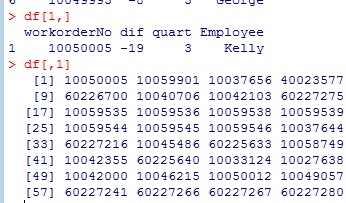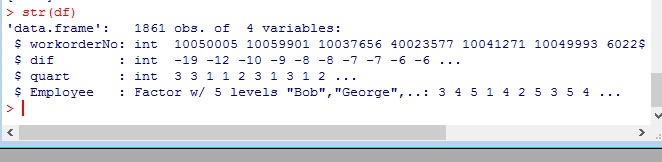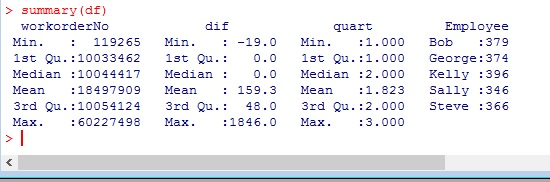# R: Working with Dataframes

At first blush, a dataframe looks a lot like a matrix. The big difference between the two is that all columns in a matrix must contain the same data type. This is not so with dataframes.

```df <- read.csv(file.choose())read.csv() automatically loads the file data into a dataframe. So without having to do anything else, we have a dataframe.

You call on data in a dataframe just like you do with a matrix.

```df[1,]
df[,1]```Using the structure function, str(), we get a look at how R configured our dataframe

`str(df)`str(df) tells us we have 1861 objects of 4 variables. – this means 1861 rows with 4 columns each.

It then goes on to identify the columns and their data. Note the Employee column. Instead of an integer like the other columns, Employee is classified as a Factor with 5 levels – meaning there are 5 unique names in this data set.

`summary(df)`summary() gives you basic stats (min, 1st Quantile, Mean, Median,…) on your numeric columns. But with the Employee column it gives you the unique names in column and a count of how often they are used.